# Big data hitting the fan? Nyquist-Shannon TOOL SAMPLE can save you

## Lessons from information theorists brainboxes - in pictures

In practice, for reasons I’ll explain below, although sampling at just over twice the frequency is in theory adequate, you may well want to sample more often than this. But the huge advantage of the Nyquist-Shannon theorem is that it sets an absolute minimum.

This is all much better illustrated by a mix of diagrams and words. Imagine that you want to digitise an analogue signal whose highest frequency component is 1,000Hz. The Nyquist theorem tells us we must sample at a minimum of 2,000 times per second (twice the highest signal frequency).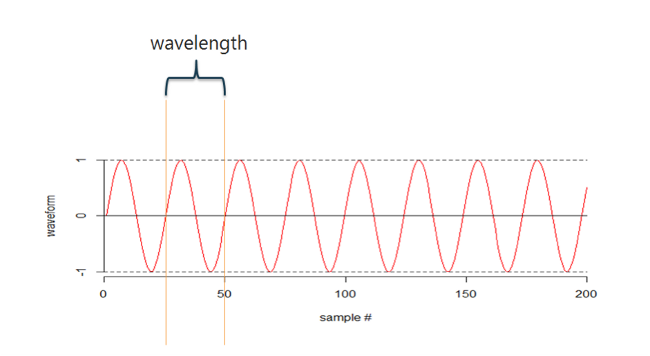Let's start by looking at a wave, below.

To illustrate why Nyquist says we need to sample at just over twice the frequency of the signal, let’s try not doing it. In the next illustration I am sampling at just under half the frequency.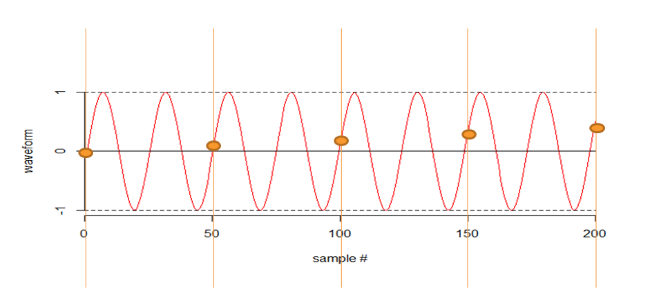My sampling “sees” a wave form of a much, much longer wave length than the original analogue one.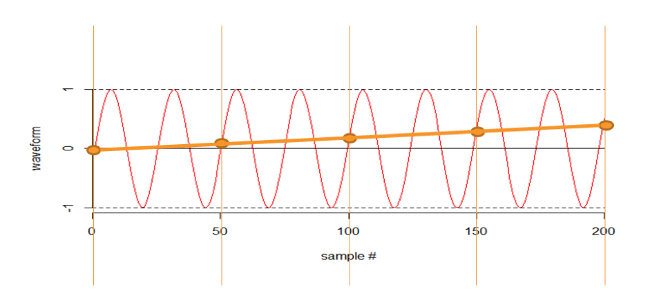OK, let’s sample more frequently. In the next illustration I am sampling at exactly twice the frequency. If I was really unlucky I would see no signal at all.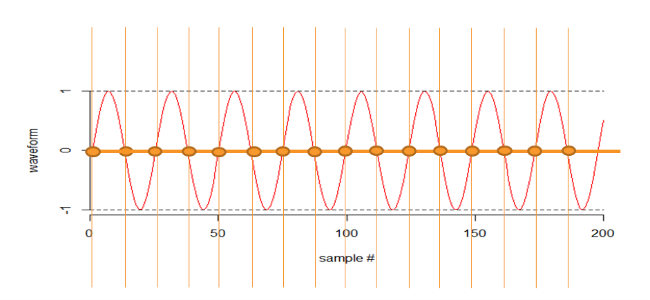If I was really lucky I would see it perfectly, below.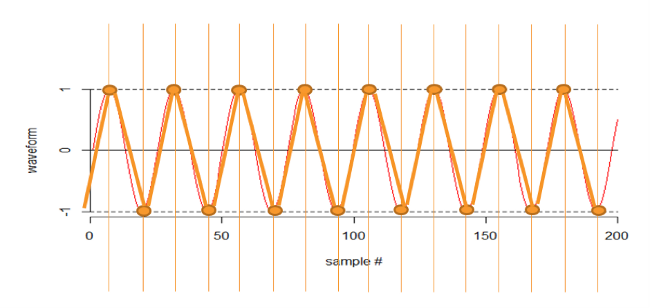And we could have anything in between, but most of the time I would detect the frequency. Here I am sampling at just over twice the frequency. This detects the frequency, but it is barely adequate (as you can see).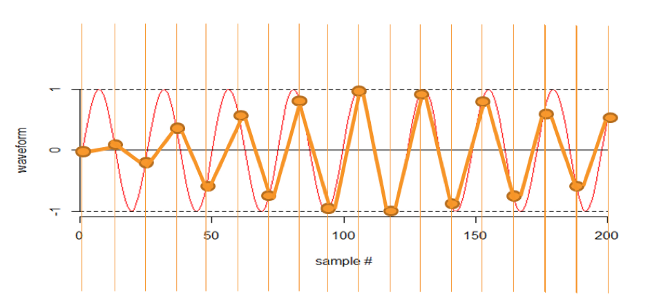Although I can try to algorithmically predict the original frequency: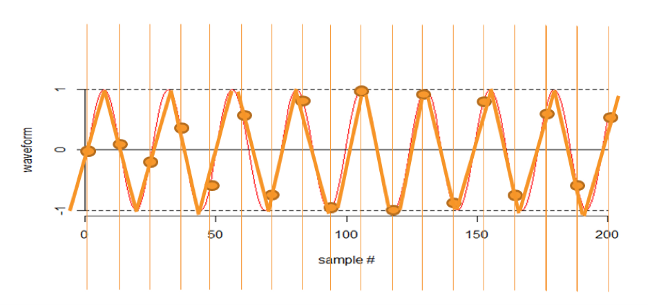In the next illustration, below, I am sampling at around eight times the frequency, which is perfectly adequate – note the scale change.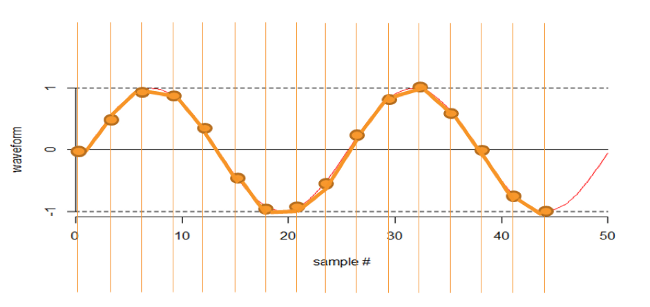So, that is the basis of the Nyquist–Shannon sampling theorem. Of course, household appliances don’t usually produce sine waves, they usually produce essentially square waves because they are either on or off. So let’s have a look at some (more or less) square waves.

If I sample at far less than the frequency, my sampling “sees” a wave form of a much, much longer wave length, as you can see in the next illustration: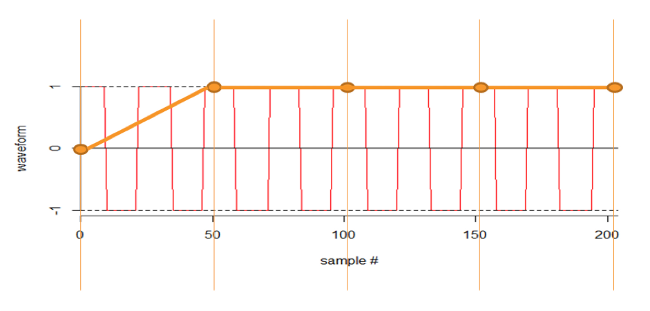Sampling at just over twice the frequency picks up the wave but loses detail: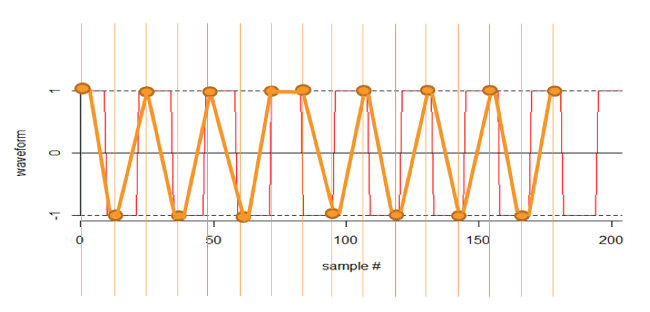Sampling at about eight times the frequency gives you what you need - note the scale change.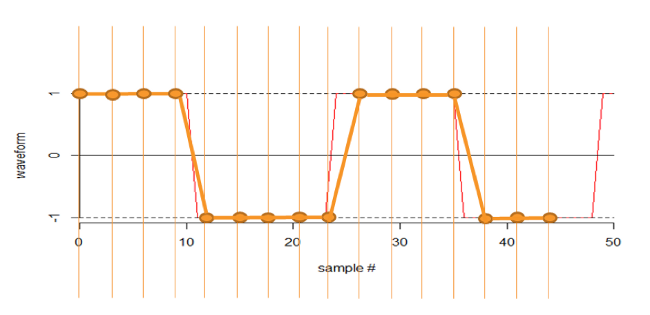So, let’s think about sampling theorem in terms of sampling power usage in a private house. The fridge turns on and off at 20-minute intervals. If you sample at one hour intervals, you essentially cannot see the fridge. If you sample at 10-minute intervals, you can see every cycle of the fridge but, in practice, you will almost certainly want to sample more frequently, perhaps every five minutes.

Nyquist–Shannon developed their theorem during the white heat of computing’s dawn – their ideas were incubated in hothouse for innovation Bell Labs.

Their principles were conceived in the world of telecommunications but are relevant to today's challenges in big data – a world where many follow the herd in hoovering up all they can and worrying about the details later.

With Nyquist–Shannon, you get the insight minus the overload. ®

Enjoyed this? See our earlier Big Five articles here and here, and look out for the final two next week.